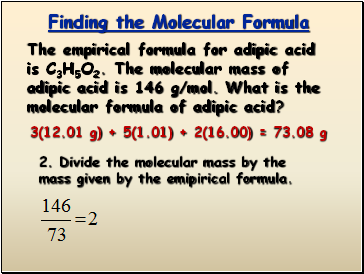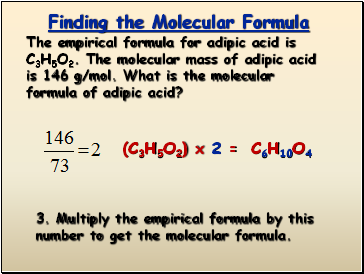# Percent Composition, Empirical and Molecular FormulasPage 2

#### WATCH ALL SLIDES

1. Find the formula mass of C3H5O2

3(12.01 g) + 5(1.01) + 2(16.00) = 73.08 g

Slide 11Finding the Molecular Formula

The empirical formula for adipic acid is C3H5O2. The molecular mass of adipic acid is 146 g/mol. What is the molecular formula of adipic acid?

3(12.01 g) + 5(1.01) + 2(16.00) = 73.08 g

2. Divide the molecular mass by the mass given by the emipirical formula.

Slide 12Finding the Molecular Formula

The empirical formula for adipic acid is C3H5O2. The molecular mass of adipic acid is 146 g/mol. What is the molecular formula of adipic acid?

3. Multiply the empirical formula by this number to get the molecular formula.

(C3H5O2) x 2 =

C6H10O4

Go to page:
1  2